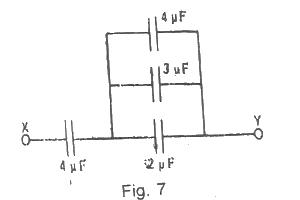# In the circuit Shown above, calculate the effective capacitance between X and Y

Question 1In the circuit Shown above, calculate the effective capacitance between X and Y